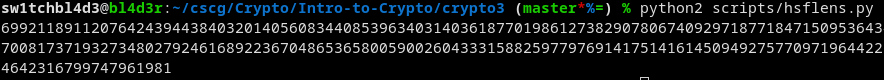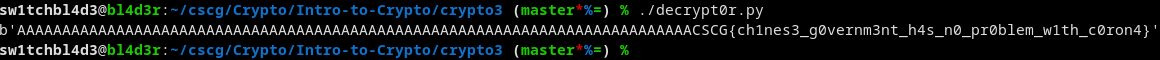# Intro to Crypto 3 - CSCG

Category: Crypto
Difficulty: Baby
Author: black-simon

## Reconnaissance

Files for you to hack along: intro-crypto1.zip

We are given 3 keys and 3 messages, they're probably the same message, just encrypted with different keys.
Looking at the keys with openssl shows that the exponent is pretty low (3).

That's what we're gonna use, a Low-Exponent-Attack.

## Exploitation

I took the script from the website and modified it so it fits my needs, like this:

```
def inverse(e, phi):
a, b, u = 0, phi, 1
while(e > 0):
q = b // e
e, a, b, u = b % e, u, e, a-q*u
if (b == 1):
return a % phi
else:
print("Must be coprime!")

def lowExponentAttack(nn, cc, e):
n, c=chineseRemainder(nn, cc)
w=nthRoot(c, e)
return w

def chineseRemainder(nn, rr):
if len(nn)==1:
return nn, rr
else:
j=len(nn)//2
m, a=chineseRemainder(nn[:j], rr[:j])
n, b=chineseRemainder(nn[j:], rr[j:])
u=inverse(m, n)
x=u*(b-a)%n*m+a
return m*n, x

def nthRoot(c, n):
p=1
while p**n<c:
p*=2
w=p
wn=w**n
while wn!=c:
p//=2
if p==0:
raise Exception("%d ist keine %d-te Potenz" %(c, n))
if wn>c:
w-=p
if wn<c:
w+=p
wn=w**n
return w

e = 3
nn = [<list of Ns>]
cc = [<list of encrypted msgs>]

print lowExponentAttack(nn, cc, e)
```

(There were a few import errors with the script they got, I just cleaned it up a bit.)

That got me this:Looks like it worked! That's the message! (in decimal form).

Converting it to text it looks like this:And there's the flag!

## Mitigation

Like I said in Crypto2, dont generate your own keys manually, with weak exponents or modulus.

Use tested tools that work.

~sw1tchbl4d3, 09/04/2020 (dd/mm/yyyy)